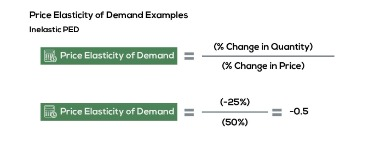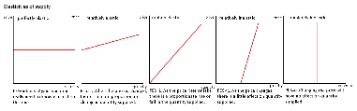# Elasticity Of Demand And Its Types

Like perfectly elastic demand, the concept of perfectly inelastic is also a theoretical concept and doesn’t find a practical application. However, the demand for necessity goods can be the closest example of perfectly inelastic demand. In a perfectly competitive market, it is assumed a firm would have a perfectly elastic demand. This is because if they increased the price, the consumers with perfect information would switch to other firms who offer the identical product. Elasticity of demand, or how much a change in price affects the quantity demanded. In this section we will dig deeper by learning how to calculate elasticity using the midpoint method. Supply can also be elastic, since a change in price will influence the quantity supplied.

A perfectly inelastic demand is the one in which there is no change measured against a price change. In other words, the elasticity of demand is the percentage change in quantity demanded divided by the percentage change in another economic variable. Different products have different price elasticities of demand. If the absolute value of the elasticity of some product is greater than one, it means that the change in the quantity demanded is greater than the change in price. This indicates a larger reaction to price change, which we describe as elastic. In perfectly elastic demand, even a small rise in price can result in a fall in demand of the good to zero, whereas a small decline in the price can increase the demand to infinity. Elasticity of Demand, or Demand Elasticity, is the measure of change in quantity demanded of a product in response to a change in any of the market variables, like price, income etc.Demand is price elastic if a change in price causes a bigger percentage change in demand. The demand curve for unitary elastic demand is represented as a rectangular hyperbola. The numerical value of relatively inelastic demand always comes out as less than 1 and the demand curve is rapidly sloping for such type of demand. Unlike the aforementioned types of demand, relatively elastic demand has a practical application as many goods respond in the same manner when there is a price change. The demand for a product can be elastic, inelastic, or unitary, depending on the rate of change in the demand with respect to the change in the price of a product.

## Relatively Inelastic Demand

When there is a sharp rise or fall due to a change in the price of the commodity, it is said to be perfectly elastic demand. An inelastic demand is one that shows a very little fluctuation in the quantity demanded with respect to a change in another economic variable. In a relatively inelastic demand, the proportionate change in the quantity demanded for a product is always less than the proportionate change in the price. The demand curve for a perfectly inelastic demand is a vertical line i.e. the slope of the curve is zero. An elastic demand is one that shows a larger fluctuation in the quantity demanded of a product, in response to even a little change in another economic variable.Also, if flights increase, people may not be able to afford it and will choose to take their holiday in the UK.

The data above indicate that the demand for cigarettes by teenagers, minority, low income and casual smokers is relatively inelastic. Addicted adult smokers, though, are even less sensitive to changes in the price—most are willing to pay whatever it takes to support their smoking habit. We can say that their demand is even more inelastic than low income or casual smokers.

## Tax On A Good With Elastic Demand

The degree of elasticity of demand helps to define the slope and shape of the demand curve. Therefore, we can determine the elasticity of demand by looking at the slope of the demand curve.

• The effect of change in economic variables is not always the same on the quantity demanded for a product.
• This indicates a larger reaction to price change, which we describe as elastic.
• The data above indicate that the demand for cigarettes by teenagers, minority, low income and casual smokers is relatively inelastic.
• In other words, if a firm increased the price by 1%, it would see all its demand evaporate.

There are several factors that affect the quantity demanded for a product such as the income levels of people, price of the product, price of other products in the segment, and various others. If demand is perfectly elastic, it means that at a certain price demand is infinite . In other words, if a firm increased the price by 1%, it would see all its demand evaporate. If demand is perfectly elastic, then demand will be horizontal.

The income levels of consumers play an important role in the quantity demanded for a product. This can be understood by looking at the difference in goods sold in the rural markets versus the goods sold in metro cities. Any change in the price of a commodity, whether it’s a decrease or increase, affects the quantity demanded for a product. For example, when there is a rise in the prices of ceiling fans, the quantity demanded goes down.

It measures the shift in demand when other economic factors change. When the proportionate change in the quantity demanded for a product is equal to the proportionate change in the price of the commodity, it is said to be unitary elastic demand. However, perfectly elastic demand is a total theoretical concept and doesn’t find a real application, unless the market is perfectly competitive and the product is homogenous.

## Calculating Elasticity

Thus, the slope of the demand curve for a perfectly elastic demand is horizontal. The result obtained from this formula determines the intensity of the effect of price change on the quantity demanded for a commodity. This measure of responsiveness of quantity demanded when there is a change in price is termed as the Price Elasticity of Demand . For example, if the price of a good goes down by 10%, the proportionate change in its demand will not go beyond 9.9..%, if it reaches 10% then it would be called unitary elastic demand. In relatively elastic demand, if the price of a good increases by 25% then the demand for the product will necessarily fall by more than 25%. The effect of change in economic variables is not always the same on the quantity demanded for a product.

These three types of Elasticity of Demand measure the sensitivity of quantity demanded to a change in the price of the good, income of consumers buying the good, and the price of another good. In a market where there is an oligopoly, multiple players compete. Thus, the quantity demanded for a product does not only depend on itself but rather, there is an effect even when prices of other goods change.Unitary elasticity is one in which the fluctuation in one variable and quantity demanded is equal. On the basis of the amount of fluctuation shown in the quantity demanded of a good, it is termed as ‘elastic’, ‘inelastic’, and ‘unitary’. With elastic demand, a tax will cause only a small rise in price. In this module you will often be asked to calculate the percentage change in the quantity. Keep in mind that this is same as the the growth rate of the quantity. As you work through the course and find other applications for calculate growth rates, you will be well prepared.

## Important Marketing Goals That Companies Must Know

Similarly, if you are buying potatoes from Covent garden, it is easy to check prices. Therefore, if a farmer increases price above the equilibrium, demand will fall significantly meaning demand is very elastic. Therefore, if one firm increased the price of dollars, above market equilibrium – no one would buy from that firm. Dairy milk chocolate bar – there are many other types of chocolate bars to choose from. Note – if chocolate in general increased in price, demand would be inelastic, but for particular types of chocolate demand is elastic.

## Elasticity Of Demand And Its Types

Luxury goods which are a high percentage of income will be more sensitive to price. If consumers have many alternatives, demand will be more sensitive to price. For example, if the price of one type of mineral water increases, people can easily switch to other brands. The demand curve of relatively elastic demand is gradually sloping. Elasticity is a concept in economics that talks about the effect of change in one economic variable on the other. We can further classify these elastic and inelastic types of demand into five categories.

## Elasticity

Relatively elastic demand refers to the demand when the proportionate change in the demand is greater than the proportionate change in the price of the good. The numerical value of relatively elastic demand ranges between one to infinity.

For example, if there is a hike of \$0.5 in the price of a cup of coffee, there are very high chances of a steep decline in the quantity demanded. Elasticity of Demand, on the other hand, specifically measures the effect of change in an economic variable on the quantity demanded of a product.

The result obtained for a substitute good would always come out to be positive as whenever there is a rise in the price of a good, the demand for its substitute rises. Whereas, the result will be negative for a complementary good. The result obtained from this formula helps to determine whether a good is a necessity good or a luxury good. Goods which are price elastic will tend to have some or all of the following characteristics. Watch this video carefully to understand how to solve for elasticity and to see what the numerical values for elasticity mean when applied to economic situations. An increase in the price of flights from London to Paris would encourage people to take the Channel Tunnel.

Posted on Posted on: 21.09.2023Categories Business Accounting Blog